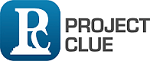We're Ready, Call Us

# HOW TO DETERMINE A RELIABLE SAMPLE SIZE FOR YOUR RESEARCH WORK

June 17, 2015. Projectclue writers

During research works, most researchers or project students find it difficult in calculating the adequate sample size to be used for their project, research or survey work. The determination of sample sizes comes up in the before questionnaires are drafted and sent into the field to get data for analysis. Miscalculation of sample sizes may lead to outright cancellation of research/project work by project supervisors as conclusions reached from the uncalculated sample size would not be precise enough in making a decision on the hypothesis under study.

The essence of adequate calculation of sample sizes is so that the conclusions reached after analysis can be generalized to the entire population under study.

As a result of our love for research, we have decided to teach students and researchers on the most reliable and effective way of calculating sample size for their project and research works. Below is one of the generally accepted methods in sample size calculation

THE TARO YAMANE METHOD

Taro Yamane , a mathematical statistician developed a statistical formular for calculating or determination of sample size in relation to the population under study so that inferences and conclusions reached after the survey can be generalized to the entire population from which the sample was gotten.

The Taro Yamane statistical formular is thus:

n =     N/ 1+ N(e)2

In the formular above;

n is the required sample size from the population under study

N is the whole population that is under study

e is the precision or sampling error which is usually 0.10,0.05 or 0.01

Example:

Using the Taro Yamane’s statistical formular to determine the adequate sample size of say 300 respondents under study. This would hence be

n =     N/ 1+ N(e)2

N=300; e= 0.1; e2= 0.01

n =     300/1+ 300(0.1)2

n= 100.

Therefore, a sample size 100 respondents out of the entire population of 300 respondents would therefore be the lowest acceptable number of responses to maintain a 95% confidence level.